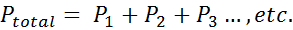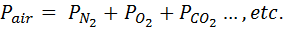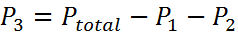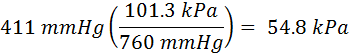# Dalton's Law

Back to Gas Laws

### Dalton's Law

The lesson is incomplete,
but the practice problems work.
Push the Green button on the right.

Dalton's Law of partial pressures basically says that the total pressure of a gas is equal to the sum of all of the partial pressures of the gases it contains. This is generally shown with the equation below:As an example, the pressure of the air making up our atmosphere can be seen as the sum of the parts of normal air, such as nitrogen, oxygen, carbon dioxide, etc. This would be written like:While the total can be calculated from the sum of the parts, it is also possible to calculate the partial pressure of a missing part if the total pressure is known. For instance, suppose a mixture is composed of three gases. If you know the total pressure and the partial pressure of two gases, you can calculate the partial pressure of the third gas by re-arranging the equation to be:It is important to note that all pressures must have the same units. If one or more of the partial pressures is in different units, they must all be converted into common units before they can be manipulated (added or subracted).

Remember that a conversion factor can be created by combining two sets of units that represent the same value. For instance, one standard atmosphere (1 atm) is the same as 760 mmHg, which is the same as 101.3 kPa, which is the same as 29.92 inHg, ans so on.

To convert the pressure 411 mmHg into kiloPascals (kPa) you would perform the following calculations:The ratio in parenthesis is one atmosphere in each of two different units. In this example, the units 'mmHg' cancel out and leave only the units of kPa.

#### Practice

Click on the image above to go go to a site where you can practice Dalton's Law probems in an infinite number of combinations

### John Dalton and his Gas Laws

John Dalton is more famously known by high school chemistry students for his atomic theory; however, in the late 1700s and early 1800's he also did considerable work with gases.

(to be continued)#### Worksheets

Here are some worksheets with problems about gas laws.#### PowerPoints

Here you can find some PowerPoints covering gas laws.#### External Videos

There are a few sources of information about gas laws on the internet. Here are a few of them